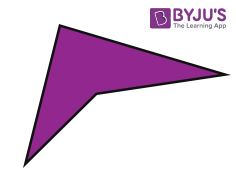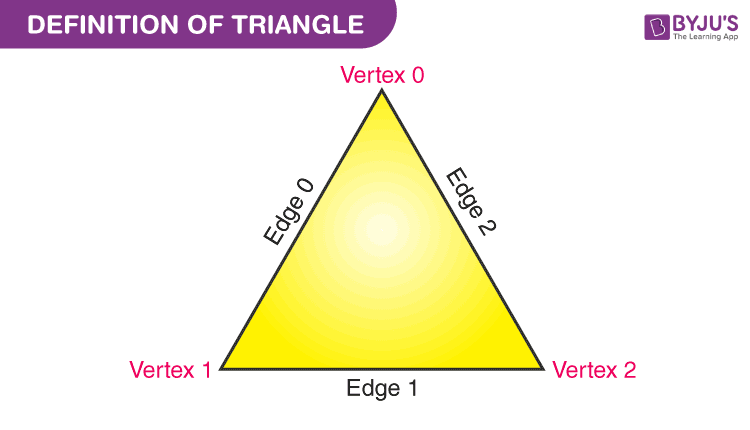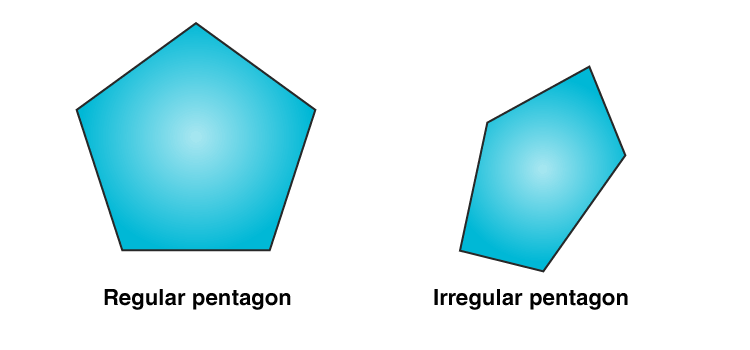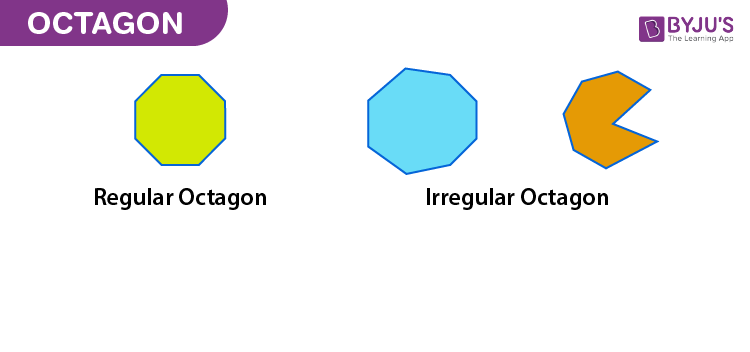# Name each polygon given below (a) Quadrilateral (b) Triangle (c) Pentagon (d) Octagon

Below are some instances of various polygons and their names.

A quadrilateral is a plane figure that has four sides or edges, and also have four corners or vertices. Quadrilaterals will typically be of standard shapes with four sides like rectangle, square, trapezoid, and kite or irregular and uncharacterized as shown below:## (b) Triangle

In Geometry, a triangle is a three-sided polygon that consists of three edges and three vertices. The most important property of a triangle is that the sum of the internal angles of a triangle is equal to 180 degrees.## (c) Pentagon

A pentagon is a polygon with 5 sides and 5 angles. The word “pentagon” is made up of two words, namely Penta and Gonia, which means five angles. All the sides of a pentagon meet with each other end to end to form a shape. Therefore, the number of sides of a pentagon is 5.## (d) Octagon

Octagon is a geometrical shape in a two-dimensional plane. The point which defines it as different from other geometrical shapes is that it has 8 sides and 8 angles.(1)(1)